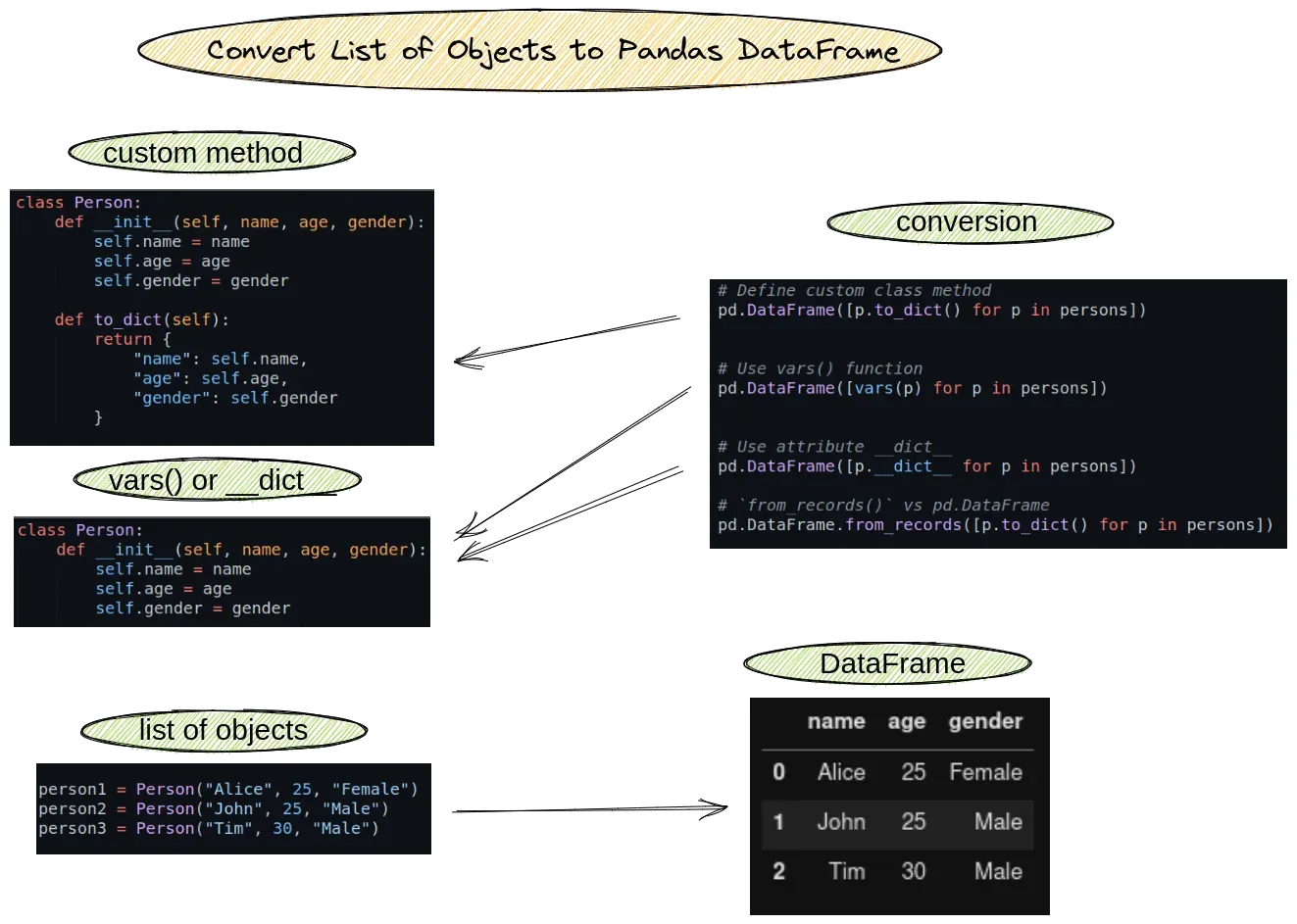To convert a list of objects to a Pandas DataFrame, we can use the:

• `pd.DataFrame` constructor
• method `from_records()`

and list comprehension:

(1) Define custom class method

``````pd.DataFrame([p.to_dict() for p in persons])
``````

(2) Use vars() function

``````pd.DataFrame([vars(p) for p in persons])
``````

(3) Use attribute dict

``````pd.DataFrame([p.__dict__ for p in persons])
``````

Here are the general steps you can follow:

• inspect the objects and the class definition
• convert list of objects based on the class

Let's check the steps to convert a list of objects in more detail. You can find visual summary of the article in this image:## Setup

To start, create your Python class - Person:

``````class Person:
def __init__(self, name, age, gender):
self.name = name
self.age = age
self.gender = gender

def to_dict(self):
return {
"name": self.name,
"age": self.age,
"gender": self.gender
}
``````

Let’s create the following 3 objects and list of them:

``````p1 = Person("Alice", 25, "Female")
p2 = Person("John", 25, "Male")
p3 = Person("Tim", 30, "Male")

persons = [p1, p2, p3]
``````

## 1: Convert list of objects - user method

We can convert a list of model objects to Pandas DataFrame by defining a custom method. This will avoid potential errors and it's a good practice to follow.

In this way we have full control on the conversion. We will use Python list comprehension for the conversion:

``````pd.DataFrame([p.to_dict() for p in persons])
``````

result is:

name age gender
0 Alice 25 Female
1 John 25 Male
2 Tim 30 Male

Conversion mapping can be changed from the method `to_dict()`:

``````def to_dict(self):
return {
"name": self.name,
"age": self.age,
"gender": self.gender
}
``````

## 2: attr dict - list of objects to dataframe

Sometimes we don't have control of the class. In this case we may use the Python attribute `__dict__` to convert the objects to dictionaries. Once we have a list of dictionaries we can create DataFrame.

So we use list comprehension and convert each object to dictionary:

``````pd.DataFrame([p.__dict__ for p in persons])
``````

the result is the same as before:

name age gender
0 Alice 25 Female
1 John 25 Male
2 Tim 30 Male

Disadvantages of this way are potential errors due to incorrect mapping or complex data types.

## 3: vars() - convert object list to dataframe

The Python `vars()` function returns the dict attribute of an object. So this way is pretty similar to the previous. This way is more pythonic and easier to read.

``````pd.DataFrame([vars(p) for p in persons])
``````

We got the same result.

Which one to choose is personal choice. I prefer `vars()` because I use: `len(my_list)` and not `my_list.__len__()`.

## 4: `from_records()` vs pd.DataFrame

To convert list of objects or dictionaries can also use method `from_records()`:

``````pd.DataFrame.from_records([p.to_dict() for p in persons])
``````

In the example above the usage of both will be equivalent.

The difference is the parameters for both:

The parameters for `pd.DataFrame` are limited to:

• data
• index
• columns
• dtype
• copy

While by using `from_records()` we have better control on the conversion by option `orient`:

• ‘columns’
• ‘index’
• ‘tight’

where

The “orientation” of the data. If the keys of the passed dict should be the columns of the resulting DataFrame, pass ‘columns’ (default). Otherwise if the keys should be rows, pass ‘index’. If ‘tight’, assume a dict with keys [‘index’, ‘columns’, ‘data’, ‘index_names’, ‘column_names’].

We can also create a multiindex dataFrame from a dictionary - you can read more on: How to Create DataFrame from Dictionary in Pandas?

## 5. parse objects in for loop

We can use for loop to define conversion logic:

``````rows = []
for p in persons:
row = {
"name": p.name,
"age": p.age,
"gender": p.gender
}
rows.append(row)

df = pd.DataFrame(rows)
``````

In this way we iterate over objects and extract only fields of interest for us.

## 6. JSON serializable objects

To convert JSON serializable objects to Pandas DataFrame we can use:

``````import json

json.dumps(p1)
``````

or:

``````json.dumps(person1, default=vars)
``````

which will give us:

``````'{"name": "Alice", "age": 25, "gender": "Female"}'
``````

## Conclusion

In this post, we saw how to convert a list of Python objects to Pandas DataFrame. We covered conversion with class methods and using built-in functions.

Examples with object parsing and JSON serializable objects were shown. Finally we discussed which way is better - Pandas DataFrame constructor or by `from_dict()`.